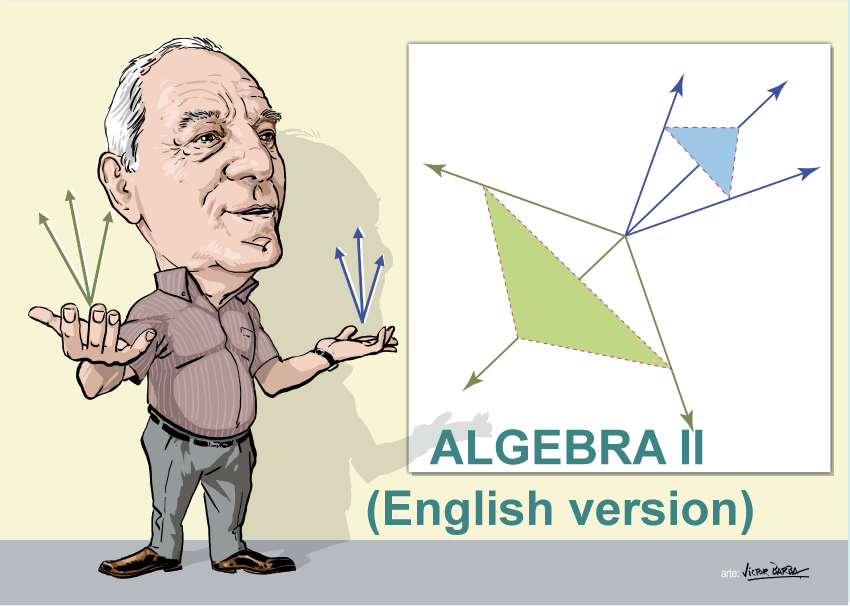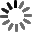El sitio web de la UCLM utiliza cookies propias y de terceros con fines técnicos y de análisis, pero no recaba ni cede datos de carácter personal de los usuarios. Sin embargo, puede haber enlaces a sitios web de terceros, con políticas de cookies distintas a la de la UCLM, que usted podrá aceptar o no cuando acceda a ellos.

Puede obtener más información en la Política de cookies. Aceptar# Algebra II (English)

Curso de fuentes OpenUCLM

We first introduce the concepts of polyhedral cone and polytope. Next, similarly to the algebra course I, an algorithm, which obtains the dual cone of a given cone and all its facets of any dimension, allows us to solve all discussed problems of linear algebra using the duality concept as a new point of view. This duality point of view allow us to solve the cone membership of a vector and the intersection of cones in a very simple way. In addition, the algorithm provides the dual cone in its simplest form, that is, as a linear space with its basis plus an acute cone with its edges. The compatibility of a linear system of inequalities is discussed and all the solutions of linear systems of inequalities are obtained. In addition, the solutions of all subsystems including all equations from the first to any of them are obtained at once. The concept of cone associated with a polytope permits us to obtain all vertices and all facets of any dimension of a polytope. The set of all feasible solutions of a linear programming problem and all its optimal solutions are obtained, and infeasibility is detected by the algorithm. Finally an example of a water supply problem is presented to show the importance of these methods in engineering design.

Autores Fecha Enrique Castillo 07/09/2019 Ingles

Me GustaIn this first block the presentation of the course will be made, exposing the block structure of the same and its sequential planning.

A course of Algebra including cones, polytopes and systems of linear inequalities. It includes some examples of engineering applications.
In this lesson we motivate the algebra course. We indicate that we use a single algorithm to deal with problems of cones, polytopes, systems of linear inequalities, obtention of facets of any dimension of cones and polytopes, etc.

In this first block the polytope and cone algebraic structures are presented, showing some illustrative examples that facilitate their understanding. The concept of a dual cone of a given cone is also presented. As a fundamental element for the development of the course, the algorithm for obtaining the dual cone of a given cone is described, which although designed to obtain the dual cone, will serve as the basis for solving all the other problems that arise in the course. It is shown that the vector space is a particular case of cone and that it is enough to add one more generator to the base in order to express the vectors of the vector space as cone, that is, generated by nonnegative linear combinations. Finally, the standard form of a cone is defined as the sum of its components of vector space and acute cone, which allows expressing the cone in its minimal form.

This lesson introduces the important concepts of polytope and cone, and the concept of dual cone of a cone. The last concept is the basis for the dual cone algorithm, that is the one used to solve all stated problems in the course.
This lesson describes the Gamma algorithm to obtain the dual cone of a cone given as a non-negative linear combination of vectors, that is, as a cone. It was proposed by Francisco Jubete and is the basis for this course, because all the problems will be solved based on this algorithm. It is surprising that only one algorithm can solve all problems dealt with in this course.
In this lesson we explain how the Gamma algorithm must be modifyied when the initial cone is given as the sum of a linear space and a cone, This occurs when the cone is given in standard form, It is shown that introducing first the linear space gnerators leads to a smaller complexity.

Standard form of a cone

Enrique Castillo

A general cone has two components, a linear space and an acute cone. In other words, a cone is the sum of these components. Of course, there are particular cones, as a linear space and an acute cone, but in general cones have the two components, In this lesson it is expalined how the standard form of acone can be obtained. In addition, the linear space is given as a basis and the acute cone as its set of edges.

This second block is focused on the algebraic applications of the algorithm, which include: the problem of the membership of a vector to a cone and the intersection of two cones. The first of these is a complex problem because it involves nonnegative linear combinations, that is, systems of linear inequations, whose solutions are not usually known by students or studied in standard algebra courses. The use of the algorithm, explained in the previous block, allows to solve the problem in a very elegant way and avoid having to solve these systems. The intersection of cones is another problem of some complexity, since it also implies the systems of inequations, solving it in an ingenious way. It can also be resolved by realizing that such an intersection is the dual cone of the dual of one of them in the other, which directly suggests how to solve it.

Vector Membership of a cone

Enrique Castillo

In this lesson we will see how to solve a complicated problem, which is whether or not a vector belongs to a cone. a simple method based on the dual cone is provided.
It is explained how the dual cone algorithm can be used to obtain the cone intersection of two cones.

This block focuses on linear inequation systems, including homogeneous and complete systems. It also explains how all the subsystems of a given system can be solved simultaneously, as well as how to analyze the compatibility of a system, that is, whether or not it has a solution.
Content

In this leasson we explain how to solve an homogeneous system of inequalities, showing that the solution is a cone, whoich is the dual of the cone generated by the coefficients of the linear equations.
In this lesson we explain how to solve a linear system of inequalities using the Gamma algorithm. It is shown that the general solution of these systems is the sum of a linear space plus a cone plus a polytope. One example of application is described in detail.
This lesson delas with the compatibility of a system of linear inequalities. These systems can have no solution, a unique solution or infinitely many solutions. An algorithm is presented that allows to know if the system has at least one solution, even in the case of the independent terms to be symbolic.

Equations of a polyhedron

Enrique Castillo

In this lesson it is explained how the equations of a polyhedron are obtained. Since we have an algorithm to solve linear systems of inequalities and we know that its solution is a polyhedron, that is, a linea space plus a cone plus a polytope, we use an inverse algorithm to obtain a linea system of inequalities having the given polyhedron as its solution. An example of application is given.
This lesson describes the alhgebraic structure of the set of all solutions of linear systems of equations and of inequations. It is very important to know these structures because it helps to understand linear suystem more deeply. The relevance of this lesson adquires the highest degree when dealing with applications, where we need to identify the boundedness of unboundedness of the solutions and how the feasible set changes with new constraints.
This lesson explains how to associate a cone to a given polytope. Since the Gamma algotithm provides the facets of all dimensions of a cone, this allows to obtain all facets of any dimension of any polytope.

In order to motivate and illustrate the power of the algorithm of orthogonalization and algebraic applications, this block is dedicated to the presentation of applications to engineering, such as water supply networks, although its application to other networks, such as of traffic, information, etc., is identical. It also includes applications to the problem of inclined planes with masses and pulleys, and electrical circuits.

An example of a water supply network is used to illustrate systems of inequalities. First a linear system of equalities models all possible alternatives for supply when the capacity of the pipes is unlimited. The solution of this problem can be obtained without any algorithm, because the dimension of the linear space in the solution is the number of holes in the network and the basis vectors and the particular solution can be easily obtained. When we limit capacities of the pipes, the solution is a polytope. Playing with the structure of this solution we decide the overdimensioned pipes, the pipes that can fail without affecting the supply, and other interesting practical questions.
An example of a company manufacturing two sets of tables is presented. The objective is to decide the number of tables to be manufactured each day to maximize benefits for a given set of constraints.
In this lesson we explain hpw the knowledge of the set of all solution permits solving linear programming problems very easily. The example of a truncated cube is used to illustrate the methods.

This block provides a series of standard exams with their solutions, to facilitate that the student can check if he has understood the material explained in the different topics of the course. Correspond to the type of exam that we have used at the University of Castilla-La Mancha for 20 years and that have been shown as very satisfactory.

Exam model 2015

Enrique Castillo

Exam model 2017

Enrique Castillo

In order to facilitate the use of the methods described in this course and students can work not only the exercises and problems raised, but other applications, we present here a computer application that implements the orthogonalization algorithm. Finally, a list of bibliographical references is given.

This video describes the application that implements the two algorithms described in the two algebra courses by Enrique Castillo.
The first one is the orthogonalization algorithm that obtains the orthogonal subspace to a given linear subspace.
The second is the dual cone algorithm, that obtains the dual cone of a given cone.

Bibliography

Enrique Castillo

Examples of data files

Enrique Castillo

Orthogonalization algorithm

Enrique Castillo

Here are all slideshow from Algebra I

Slideshows

Enrique Castillo